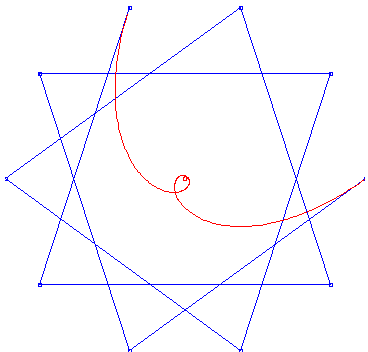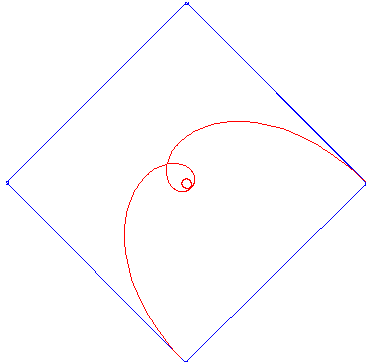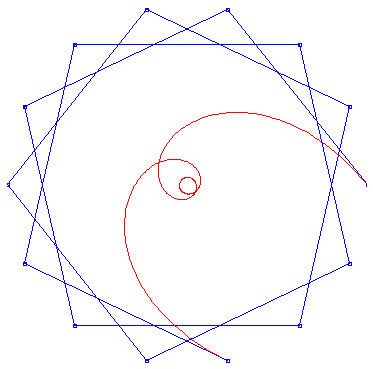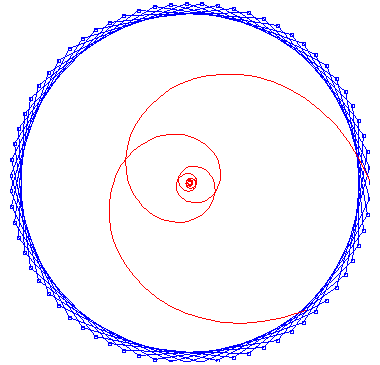# Paul Barry

## 1  Introduction

The Bezier curve is a basic element of many computer graphic toolsets. This is not surprising, as it is easy to define and has well-understood mathematical properties. In this article, we apply the Discrete Fourier Transform to the construction of Bezier curves to gain more insight into their structure. As a Bezier curve is determined by its control polygon, this analysis is intimately linked to the Fourier analysis of the control polygon. An analysis of polygons in the plane has been carried out in . Our work can be seen as a specialization of this.

## 2  Preliminaries

We let P0, P1,¼,Pn be n+1 points in the plane. Let t be a parameter, normally an element of [0,1]. Then the Bezier curved defined by the points P0, P1,¼,Pn is defined by the vector equation
 P(t) = n å k = 0 Ckn(1-t)ktn-kPi
(1)
We recall that the polygon determined by the points P0, P1,¼,Pn is called the control polygon of the curve. For instance, the line segments P0P1 and Pn-1Pn are tangents to the start, respectively, the end of the curve.

The above equation results from an iterated process of subdividing line segments in the ratio t:(1-t), starting with the line segments PkPk+1, k = 0¼n. In the case of n = 3, for instance, we have

 P(t) = (1-t)3P0+3t(1-t)2P1+3t2P2+t3P3
æ
è
 (1-t)3
 3t(1-t)2
 3t2(1-t)
 t3
ö
ø
æ
ç
ç
ç
ç
ç
è
 P0
 P1
 P2
 P3
ö
÷
÷
÷
÷
÷
ø
æ
è
 1
 t
 t2
 t3
ö
ø
æ
ç
ç
ç
ç
ç
è
 1
 0
 0
 0
 -3
 3
 0
 0
 3
 -6
 3
 0
 -1
 3
 -3
 1
ö
÷
÷
÷
÷
÷
ø
æ
ç
ç
ç
ç
ç
è
 P0
 P1
 P2
 P3
ö
÷
÷
÷
÷
÷
ø
æ
è
 1
 3t
 3t2
 t3
ö
ø
æ
ç
ç
ç
ç
ç
è
 1
 0
 0
 0
 -1
 1
 0
 0
 1
 -2
 1
 0
 -1
 3
 -3
 1
ö
÷
÷
÷
÷
÷
ø
æ
ç
ç
ç
ç
ç
è
 P0
 P1
 P2
 P3
ö
÷
÷
÷
÷
÷
ø
(2)
We recognize in the square matrix the inverse of the 4×4 binomial matrix. This is the matrix with general form
B æ
ç
ç
ç
ç
ç
ç
ç
ç
ç
ç
è
 1
 0
 0
 0
 0
 ¼
 1
 1
 0
 0
 0
 ¼
 1
 2
 1
 0
 0
 ¼
 1
 3
 3
 1
 0
 ¼
 1
 4
 6
 4
 1
 ¼
 :
 :
 :
 :
 :
 ···
ö
÷
÷
÷
÷
÷
÷
÷
÷
÷
÷
ø
whose (j,k)-th element is equal to C(j-1,k-1).

It is instructive to see the effect of B-1 on the power basis (1,x,x2,¼). In the 4×4 case, for instance, we have

æ
ç
ç
ç
ç
ç
è
 1
 0
 0
 0
 -1
 1
 0
 0
 1
 -2
 1
 0
 -1
 3
 -3
 1
ö
÷
÷
÷
÷
÷
ø
æ
ç
ç
ç
ç
ç
è
 1
 x
 x2
 x3
ö
÷
÷
÷
÷
÷
ø
æ
ç
ç
ç
ç
ç
è
 1
 x-1
 (x-1)2
 (x-1)3
ö
÷
÷
÷
÷
÷
ø
We now introduce the Discrete Fourier Transform . If we let f0, f1¼fn be n+1 numbers, real or complex, then their Discrete Fourier Transform is the set of n+1 numbers
 ^ f j = n å k = 0 fke-2pijk/(n+1)
(3)
where i = [Ö(-1)]. The numbers [^f]j are in general complex numbers. We can invert this transformation as follows :
 fk = 1 n+1 n å j = 0 ^ f j e2pijk/(n+1)
(4)
We shall refer to the (n+1)×(n+1) matrix with (j,k)-th element e-2pijk/(n+1) as the (discrete) Fourier matrix F. For instance, in the case n = 3, we have
æ
ç
ç
ç
ç
ç
ç
ç
è
 ^ f 0
 ^ f 1
 ^ f 2
 ^ f 3
ö
÷
÷
÷
÷
÷
÷
÷
ø
æ
ç
ç
ç
ç
ç
è
 1
 1
 1
 1
 1
 i
 -1
 -i
 1
 -1
 1
 -1
 1
 -i
 -1
 i
ö
÷
÷
÷
÷
÷
ø
æ
ç
ç
ç
ç
ç
è
 f0
 f1
 f2
 f3
ö
÷
÷
÷
÷
÷
ø
æ
ç
ç
ç
ç
ç
è
 f0
 f1
 f2
 f3
ö
÷
÷
÷
÷
÷
ø
1
4
æ
ç
ç
ç
ç
ç
è
 1
 1
 1
 1
 1
 -i
 -1
 i
 1
 -1
 1
 -1
 1
 i
 -1
 -i
ö
÷
÷
÷
÷
÷
ø
æ
ç
ç
ç
ç
ç
ç
ç
è
 ^ f 0
 ^ f 1
 ^ f 2
 ^ f 3
ö
÷
÷
÷
÷
÷
÷
÷
ø

## 3  Analyzing Bezier curves

We now apply the foregoing to Bezier curves in the plane. We observe that a point P in the plane can be regarded as a complex number, so that taking the Fourier transform of a set of points makes sense. We let wj = e-2pij/(n+1) be a solution of the equation
 zn+1-1 = 0
(5)
Then
 P(t) = n å k = 0 Ckntk(1-t)n-kPk
 = n å k = 0 Cknti(1-t)n-k 1 n+1 n å j = 0 ^ P j wjk
 = 1 n+1 n å k = 0 n å j = 0 Ckntkwjk(1-t)n-k ^ P j
 = 1 n+1 n å j = 0 ^ P j n å k = 0 Ckn(twj)k (1-t)n-k
 = 1 n+1 n å j = 0 ^ P j ((1-t).1+twj)n
(6)
This exhibits P(t) as a linear combination of ``basic" Bezier curves, since we have
 Bj(t) = ((1-t).1+twj)n
 = n å k = 0 Ckn tk(1-t)n-kwjk
 = n å k = 0 Ckntk(1-t)n-kwkj
(7)
Hence
 P(t) = 1 n+1 n å j = 0 ^ P j Bj(t)
(8)
for the Bezier curve with control polygon (P0,P1,¼,Pn).

These basic Bezier curves have control polygons determined by the numbers {wkj}, and hence the geometry of these curves is determined by the geometry of the corresponding star-polygons .

## 4  A worked example

We let B be the 4×4 binomial matrix :
B æ
ç
ç
ç
ç
ç
è
 1
 0
 0
 0
 1
 1
 0
 0
 1
 2
 1
 0
 1
 3
 3
 1
ö
÷
÷
÷
÷
÷
ø
Then its inverse is given by
B-1 æ
ç
ç
ç
ç
ç
è
 1
 0
 0
 0
 -1
 1
 0
 0
 1
 -2
 1
 0
 -1
 3
 -3
 1
ö
÷
÷
÷
÷
÷
ø
The 4×4 Fourier matrix F is given by
F æ
ç
ç
ç
ç
ç
è
 1
 1
 1
 1
 1
 i
 -1
 -i
 1
 -1
 1
 -1
 1
 -i
 -1
 i
ö
÷
÷
÷
÷
÷
ø
with inverse
F-1 1
4
æ
ç
ç
ç
ç
ç
è
 1
 1
 1
 1
 1
 -i
 -1
 i
 1
 -1
 1
 -1
 1
 i
 -1
 -i
ö
÷
÷
÷
÷
÷
ø
1
4
æ
ç
ç
ç
ç
ç
è
 1
 1
 1
 1
 1
 w1
 w2
 w3
 1
 w12
 w22
 w32
 1
 w13
 w23
 w33
ö
÷
÷
÷
÷
÷
ø
Then (2) says that
P(t) =  æ
è
 1
 3t
 3t2
 t3
ö
ø
B-1 æ
ç
ç
ç
ç
ç
è
 P0
 P1
 P2
 P3
ö
÷
÷
÷
÷
÷
ø
æ
è
 1
 3t
 3t2
 t3
ö
ø
B-1F-1F æ
ç
ç
ç
ç
ç
è
 P0
 P1
 P2
 P3
ö
÷
÷
÷
÷
÷
ø
æ
è
 1
 3t
 3t2
 t3
ö
ø
B-1F-1 æ
ç
ç
ç
ç
ç
ç
ç
è
 ^ P 0
 ^ P 1
 ^ P 2
 ^ P 3
ö
÷
÷
÷
÷
÷
÷
÷
ø
æ
è
 1
 3t
 3t2
 t3
ö
ø
æ
ç
ç
ç
ç
ç
è
 1
 1
 1
 1
 0
 w1-1
 w2-1
 w3-1
 0
 (w1-1)2
 (w2-1)2
 (w3-1)2
 0
 (w1-1)3
 (w2-1)3
 (w3-1)3
ö
÷
÷
÷
÷
÷
ø
æ
ç
ç
ç
ç
ç
ç
ç
è
 ^ P 0
 ^ P 1
 ^ P 2
 ^ P 3
ö
÷
÷
÷
÷
÷
÷
÷
ø
(9)
Hence
 4P(t) = ^ P0 + ^ P1 (1+t(w1-1))3+ ^ P2 (1+t(w2-1))3+ ^ P3 (1+t(w3-1))3
 = ^ P0 + ^ P1 ((1-t).1+tw1)3+ ^ P2 ((1-t).1+tw2)3+ ^ P3 ((1-t).1+tw3)3
(10)
Here, we have, for instance
 ((1-t).1+tw1)3 = (1-t)3.1+3(1-t)2tw1+3(1-t)t2w12+t3w13
(11)
which is the Bezier curve with the four roots of unity 1,w1,w12,w13 or 1, -i, -1, i as control points. We then have
 P(t) = 1 4 ( ^ P0 B0(t)+ ^ P1 B1(t)+ ^ P2 B2(t)+ ^ P3 B3(t))
(12)
where
 Bj(t) = ((1-t).1+twj)3 = 3 å k ((1-t)ktn-kwjk
(13)
with wj = e-2pij/4.

This shows that the Bezier curve P(t) is a ``weighted average'' of basic Bezier curves. We note that in the above case, the basic curve B0(t) degenerates to the single point (1,0) in the plane, while the basic curve B2(t) describes the line-segment from (1,0) to (-1,0) and back again.Basic Bezier curve j = 7, n+1 = 10Basic Bezier Curve, j = 3, n+1 = 12Basic Bezier curve, j = 10, n +1= 12Basic Bezier curve, j = 3, n+1 = 14Basic Bezier curve, j = 5, n+1 = 14Basic Bezier curve, j = 8, n +1= 71

REFERENCES

1. H.S.M. Coxeter, Introduction to Geometry (2nd ed.), Wiley, New York, 1969
2. J.C. Fisher, D. Ruoff & J. Shilleto, Perpendicular Polygons, Amer. Math. Monthly 92 (1985), 23-37
3. E. W. Weisstein, http://mathworld.wolfram.com/DiscreteFourierTransform.html/,2003